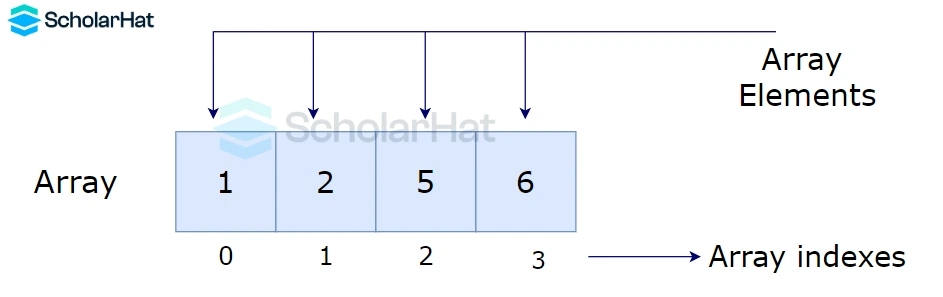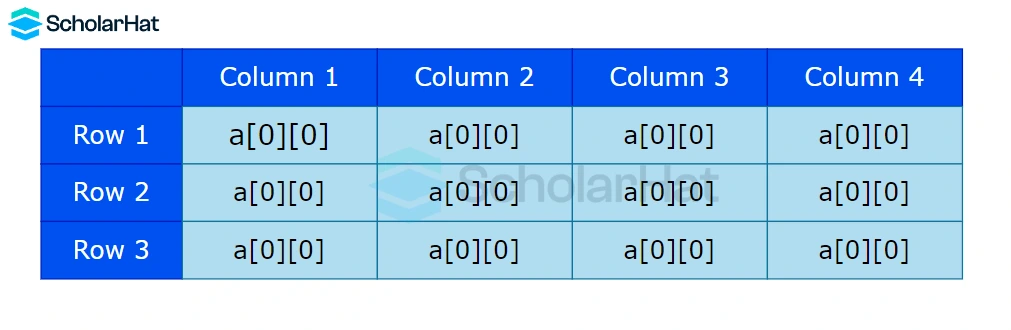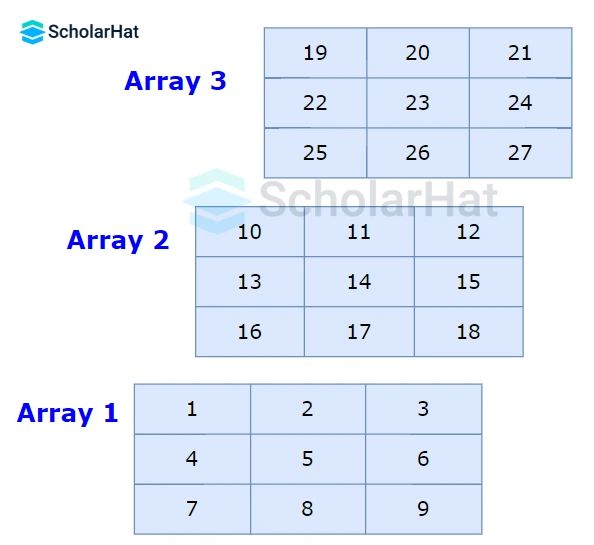# Types of Array in Data Structure

Amit Kumar Ghosh  8 min read
22 Sep 2023
Beginner
462 Views

## Types of Array in Data Structure: An Overview

Array data structures are essential components of many computer applications. It’s important for anyone interested in computer science and software engineering to become familiar with the different types of arrays available, as well as how they can be used in problem-solving strategies. This article will introduce readers to key concepts related to Data Structure and analysis (DSA) and array data structures, giving an overview of different types of arrays along with their associated uses, benefits, and types of array in data structure with examples and challenges when programming solutions. Readers will gain a better understanding of the differences between one-dimensional and multi-dimensional arrays while learning how each array type is effectively combined into complex programming solutions.

## Types of Array in Data Structure

In computer programming, an array is a data structure that stores a collection of elements of the same type. There are several types of Arrays in data structure Those are:

### One-dimensional array:

A one-dimensional array, also known as a vector, is the simplest type of array. It consists of a single row or column of elements and is accessed using a single index.#### Example

``````int myArray = {1, 2, 3, 4, 5};
``````

### Two-dimensional array:

A two-dimensional array, also known as a matrix, is an array of arrays. It consists of a grid of elements that can be accessed using two indices.#### Example

``````int myArray = {{1, 2, 3}, {4, 5, 6}, {7, 8, 9}};
``````

### Multi-dimensional array:

A multi-dimensional array is an array with more than two dimensions. It can be thought of as an array of arrays of arrays, and so on.#### Example

``````int myArray = {{{1, 2, 3}, {4, 5, 6}, {7, 8, 9}}, {{10, 11, 12}, {13, 14, 15}, {16, 17, 18}}, {{19, 20, 21}, {22, 23, 24}, {25, 26, 27}}};
``````

### Jagged array:

A jagged array is an array of arrays, where each sub-array can have a different number of elements.

#### Example

``int myArray[][] = {{1, 2, 3}, {4, 5}, {6, 7, 8, 9}};``

## Operations of the array in data structure

There are some operations of the array in the data structure:
• Insertion - It is used to add an element at a particular index.
• Deletion - It is used to delete an element from a particular index.
• Search - It is used to search an element using the given index or by the value.
• Update - It updates an element at a particular index.

### Array Insertion

Insert an element in an array is a basic operation performed on an array. Inserting is the process of adding another element into the array at the beginning, end, or at the specified index position. The following algorithm inserts an element to the kth position of the array A with n elements.

#### insert(A, n, k, element)

Step1: Set j:=n.
Step 2: Repeat steps 3 and 4 while j>=k.
Step 3: Set A[j+1] := A[j].
Step 4: Set j:=j+1.
[end of the Repeat loop]
Step 5: Set A[k]:= element.
Step 6: Set n:=n+1
Step 7: Stop

#### Output

Array elements before insertion
[18, 30, 15, 70, 12]
Array elements after insertion
[18, 30, 15, 50, 70, 12]

### Array Deletion

Delete element from array is the process of removing an element from the array. This can be done from the specified position as in the case of deletion.

#### Algorithm

Step 1: Start
Step 2: [Initialize counter variable. ] Set i = pos - 1
Step 3: Repeat Steps 04 and 05 for i = pos - 1 to i < size
Step 4: [Move ith element backward (left). ] set a[i] = a[i+1]
Step 5: [Increase counter. ] Set i = i + 1
Step 6: [End of step 03 loop. ]
Step 7: [Reset the size of the array. ] set size = size - 1
Step 8: Stop

#### Output

Given array elements are :
arr = 18, arr = 30, arr = 15, arr = 70, arr = 12,
Elements of array after deletion:
arr = 18, arr = 15, arr = 70, arr = 12,

### Array Search operation

Searching an array means finding the index of a specific element within the array. The search operation is performed by iterating over the array elements, comparing each element with the target element, and returning the index of the target element if found. If the target element is not found, then the search operation returns a failure signal (e.g., -1 or None).

#### Algorithm:

Step 1: Initialize the array with elements.
Step 2: Initialize the element to be searched.
Step 3: Loop through the array and compare each element with the element to be searched.
Step 4:If the element is found, return the index of the element.

#### Output

Given array elements are:
arr = 18, arr = 30, arr = 15, arr = 70, arr = 12,
Element to be searched = 70
Element 70 is found at the 4 position

## FAQs

### 1. What are the different types of arrays?

One-dimensional, two-dimensional, multi-dimensional, and jagged arrays are some of the different types of arrays.

### 2. What is a 3-dimensional array in data structure?

An array having three dimensions that form a cube of elements is referred to as a 3-dimensional array in data structures.

### 3. What are the two types of multidimensional arrays?

Rectangular arrays & jagged arrays are the two varieties of multidimensional arrays.

### 4. What is a multidimensional array?

An array with more than one dimension, such as a matrix or cube, is referred to as a multidimensional array.

### 5. What are the 4 types of arrays?

One-dimensional, two-dimensional, multi-dimensional, and jagged arrays are the four different forms of arrays.
##### Summary
This article provides an introduction to array data structures, which are essential in computer applications. It describes the usage and programming challenges of one-dimensional, two-dimensional, multi-dimensional, and jagged arrays. It dives into array operations such as insertion, deletion, and search, as well as the methods used for each. Understanding these ideas allows readers to efficiently use arrays in problem-solving, making it a must-read for computer science and software engineering fans.
Similar Articles